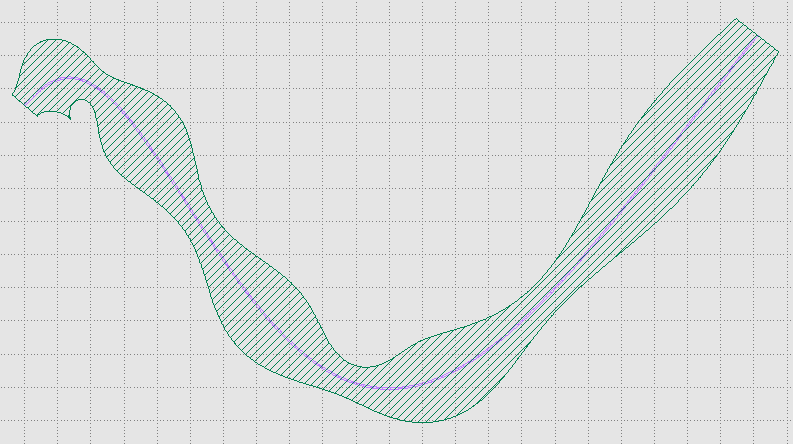Home Forums Nazca Questions and Answers viper() method Reply To: viper() method

#5524

Dear Marek,

The viper can be found in util.py and can be accessed follows

``````import nazca as nd
nd.util.viper()``````

The viper() is a “lower level” function on top of polyline2polygon(). The latter has been upgraded to handle variable widths better. The viper returns an array of points, which can be fed into a Polygon. Below is an example where x, y are the coordinates that the viper follows and w the width.

``````import nazca as nd
import math as m

def x(t):
return (2+15*t)*m.exp(t)

def y(t):
return (2+20*t)*m.cos(t*5)

def w(t):
return 2+3*(m.sin(t*15))**2

# N is the number of points, x, y, w functions of one parameter.
xygon = nd.util.viper(x, y, w, N=200)
nd.Polygon(points=xygon).put(0, 5)

# draw the same viper spine again, but with constant width
xygon = nd.util.viper(x, y, lambda t: 0.1, N=200)
nd.Polygon(points=xygon, layer=2).put(0, 5)

nd.export_gds()``````For the viper to also become an interconnect it would need some extra checks on how many polygon points are needed for grid snapping at the right resolution. In the present case just give a sufficiently large N.

Ronald